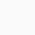# Engineering Calculus and Differential Equations

Learn fundamental concepts of single-variable calculus and ordinary differential equations, as well as their applications in engineering fields. Apply mathematical skills to model and solve engineering problems.

How do electrical engineers find out all the currents and voltages in a network of connected components? How do civil engineers calculate the materials necessary to construct a curved dome over a new sports arena? How do space flight engineers launch an exploratory probe?

If questions like these pique your interest, this course is for you!

Calculus with differential equations is the universal language of engineers. In this course, “Engineering Calculus and Differential Equations,” we will introduce fundamental concepts of single-variable calculus and ordinary differential equations. We’ll explore their applications in different engineering fields. In particular, you will learn how to apply mathematical skills to model and solve real engineering problems.

This course will enable you to develop a more profound understanding of engineering concepts and enhance your skills in solving engineering problems. In other words, you will be able to construct relatively simple models of change and deduce their consequences. By studying these, you will learn how to monitor and even control a given system to do what you want it to do.

Techniques widely used in engineering will be illustrated; such as Laplace transform for solving problems in vibrations and signal processing. We have designed animations and interactive visualizations to supplement complex mathematical theories and facilitate understanding of the dynamic nature of topics involving calculus.

• Limits, Continuity, and differentiation in engineering Calculus
• Applications of Derivatives
• Parametric Equations and Polar Coordinates
• Techniques of Integration
• Applications of Definite Integrals
• Engineering Differential Equations and First Order Equations
• Homogeneous, Inhomogeneous Equations, and Exact Equations
• Homogeneous Linear Equations with Constant Coefficients
• Cauchy-Euler Equations andLaplace Transforms
• How to analyze a given engineering problem
• Ways to identify appropriate calculus and ordinary differential equations (ODE) skills
• How to formulate the mathematical problem
• How to find the solutions for engineering problems
Engineering Calculus and Differential Equations

Originally posted 2022-01-25 09:55:30.

-1

We will be happy to hear your thoughts